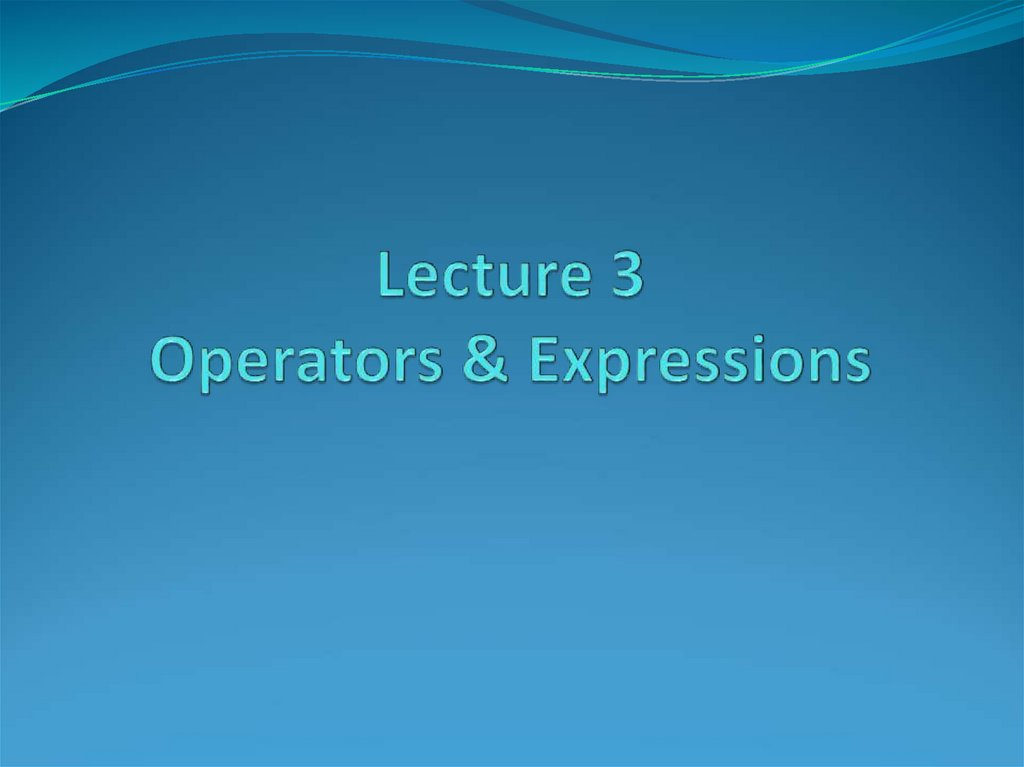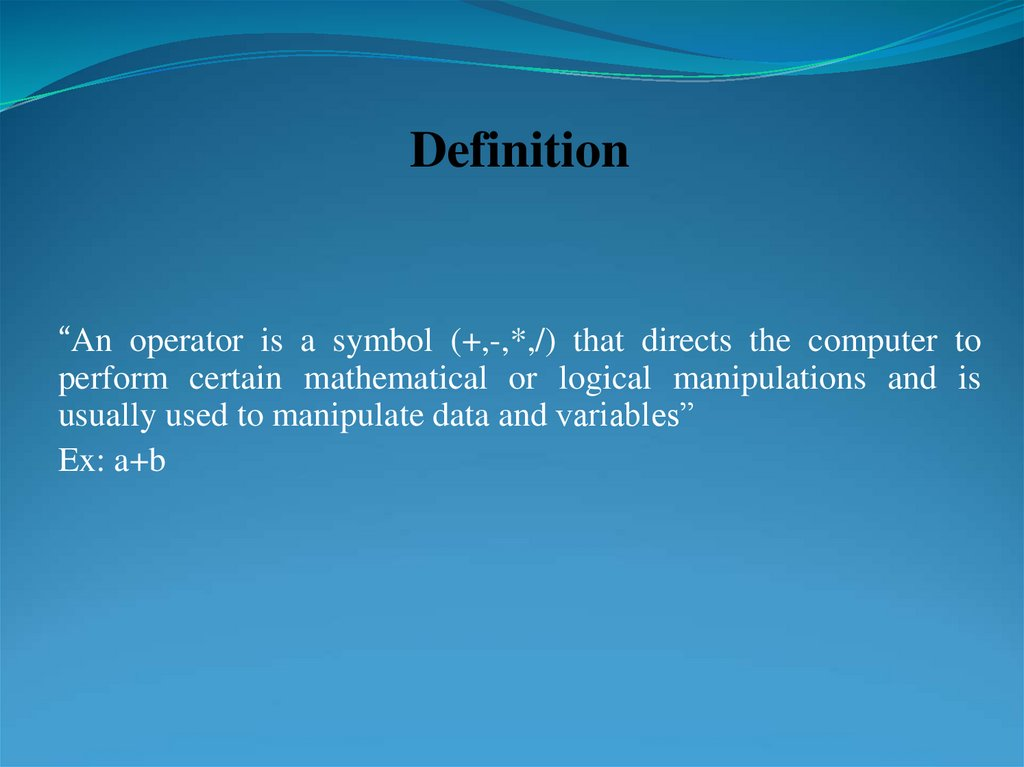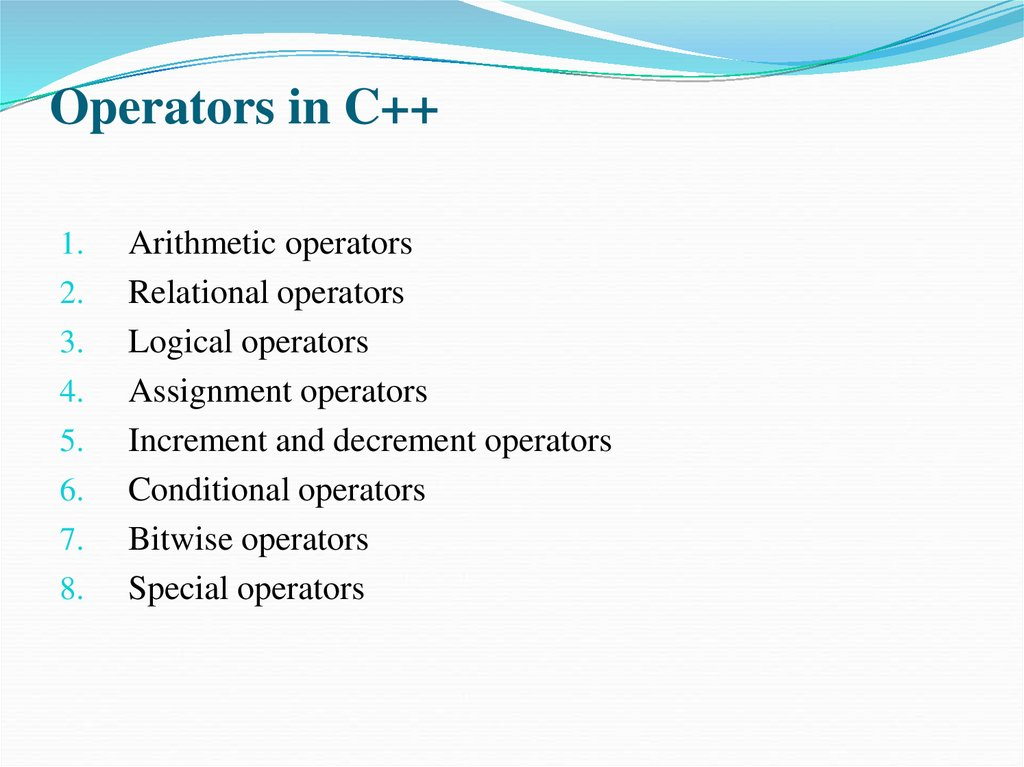# Operators & Expressions. Lecture 3

## 2.

Definition
“An operator is a symbol (+,-,*,/) that directs the computer to
perform certain mathematical or logical manipulations and is
usually used to manipulate data and variables”
Ex: a+b

## 3. Operators in C++

1.
2.
3.
4.
5.
6.
7.
8.
Arithmetic operators
Relational operators
Logical operators
Assignment operators
Increment and decrement operators
Conditional operators
Bitwise operators
Special operators

Operation
Operator
Syntax
Result
+
a+b
12
Subtraction
-
a–b
6
Multiply
*
a*b
27
Divide
/
a/b
3
Modulus
%
a%b
0

## 5. Relational Operators

Operator
Meaning
<
Is less than
<=
Is less than or equal to
>
Is greater than
>=
Is greater than or equal to
==
Equal to
!=
Not equal to

## 6.

Logical Operators
Operator
&&
Meaning
Logical AND
||
Logical OR
!
Logical NOT
Logical expression
expression-
or
a
compound
relational
An expression that combines two or more relational
expressions
Ex: if (a==b && b==c)

## 7. Truth Table

Value of the expression
a
b
a && b
a || b
0
0
0
0
0
1
0
1
1
0
0
1
1
1
1
1

## 8. Assignment Operator

Syntax:
v op = exp;
Where v = variable,
op = shorthand assignment operator
exp = expression
Ex: x=x+3
x+=3

## 9. Shorthand Assignment Operators

Simple Assignment operator
Shorthand Operator
a = a+1
a + =1
a = a-1
a - =1
a = a* (m+n)
a*=m+n
a = a / (m+n)
a/=m+n
a = a %b
a %=b

## 10. Increment & Decrement Operators

Increment & Decrement Operators
C++ supports 2 useful operators namely
1. Increment ++
2. Decrement- -operators
The ++ operator adds a value 1 to the operand
The -- operator subtracts 1 from the operand
++a or a++
--a or a--

## 11. Rules for ++ & -- Operators

Rules for ++ & -- Operators
These require variables as their operands.
When postfix either ++ or -- is used with the variable in a given
expression, the expression is evaluated first and then it is
incremented or decremented by one.
When prefix either ++ or – is used with the variable in a given
expression, it is incremented or decremented by .one first and
then the expression is evaluated with the new value

## 12. Examples for ++ and -- Operators

Let the value of a =5 and b=++a then
a = b =6
Let the value of a = 5 and b=a++ then
a =6 but b=5
i.e.:
1. a prefix operator first adds 1 to the operand and then the result is
assigned to the variable on the left
2. a postfix operator first assigns the value to the variable on left
and then increments the operand.

## 13. Conditional Operators

Syntax:
exp1 ? exp2 : exp3
Where exp1,exp2 and exp3 are expressions
Working of the ? Operator:
Exp1 is evaluated first, if it is nonzero(1/true) then the expression2
is evaluated and this becomes the value of the expression,
If exp1 is false(0/zero) exp3 is evaluated and its value becomes the
value of the expression
Ex: m=2;
n=3
r=(m>n) ? m : n;

## 14. Bitwise Operators

These operators allow manipulation of data at the bit level
Operator
&
|
^
<<
>>
Meaning
Bitwise AND
Bitwise OR
Bitwise exclusive OR
Shift left
Shift right

## 15. Special Operators

1.
2.
3.
4.
Comma operator ( ,)
sizeof operator – sizeof( )
Pointer operators – ( & and *)
Member selection operators – ( . and ->)

## 16. Arithmetic Expressions

Algebraic expression
C expression
axb-c
a*b-c
(m+n)(x+y)
(m+n)*(x+y)
ab
c
3x2+2x+1
a*b/c
3*x*x+2*x+1
a/b
a
b
S=
a b c
2
S=(a+b+c)/2

## 17. Arithmetic Expressions

Algebraic expression
s(s a)(s b)(s c)
area=
b
2
2
a b
Sin
y
2
1 x
xy
2
y
2
1 x
xy
2
2
y
x
sin
C expression
area=sqrt(s*(s-a)*(s-b)*(s-c))
sin(b/sqrt(a*a+b*b))
tow1=sqrt((rowx-rowy)/2+tow*x*y*y)
tow1=sqrt(pow((rowx-rowy)/2,2)+tow*x*y*y)
y=(alpha+beta)/sin(theta*3.1416/180)+abs(x)

## 18. Precedence of operators

BODMAS RULEBrackets of Division Multiplication Addition Subtraction
Brackets will have the highest precedence and have to be
evaluated first, then comes of , then comes
division, multiplication, addition and finally subtraction.
C language uses some rules in evaluating the expressions
and they r called as precedence rules or sometimes also
referred to as hierarchy of operations, with some operators
with highest precedence and some with least.
The 2 distinct priority levels of arithmetic operators in c areHighest priority : * / %
Lowest priority : + -

## 19. Rules for Evaluation of Expression

1.
2.
3.
4.
5.
6.
First parenthesized sub expression from left to right are
evaluated.
If parentheses are nested, the evaluation begins with the
innermost sub expression
The precedence rule is applied in determining the order of
application of operators in evaluating sub expressions
The associatively rule is applied when 2 or more operators
of the same precedence level appear in a sub expression.
Arithmetic expressions are evaluated from left to right using
the rules of precedence
When parentheses are used, the expressions within
parentheses assume highest priority

## 20. Hierarchy of operators

Operator
Description
Associativity
( ), [ ]
Function call, array
element reference
Left to Right
+, -, ++, - ,!,~,*,&
Unary plus, minus,
increment, decrement,
logical negation, 1’s
complement, pointer
*, / , %
Multiplication,
division, modulus
Right to Left
Left to Right

## 21. Example 1

Evaluate x1=(-b+ sqrt (b*b-4*a*c))/(2*a) @ a=1, b=-5, c=6
=(-(-5)+sqrt((-5)(-5)-4*1*6))/(2*1)
=(5 + sqrt((-5)(-5)-4*1*6))/(2*1)
=(5 + sqrt(25 -4*1*6))/(2*1)
=(5 + sqrt(25 -4*6))/(2*1)
=(5 + sqrt(25 -24))/(2*1)
=(5 + sqrt(1))/(2*1)
=(5 + 1.0)/(2*1)
=(6.0)/(2*1)
=6.0/2 = 3.0

## 22. Example 2

Evaluate the expression when a=4
b=a- ++a
=a – 5
=5-5
=0